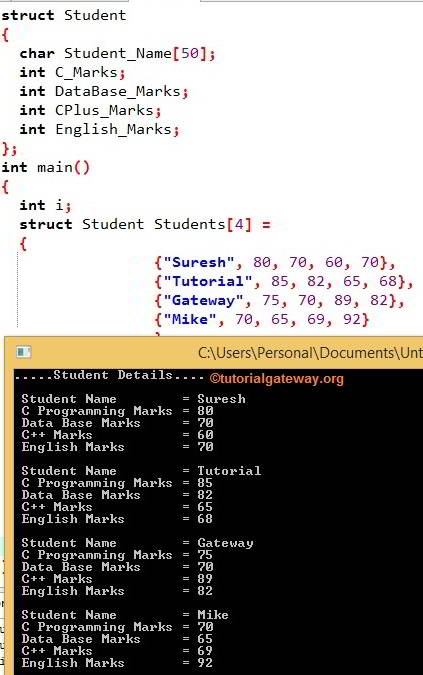# Array of Structures in C

The array of Structures in C Programming: Structures are useful for grouping different data types to organize the data in a structural way. And Arrays are used to group the same data type values. In this article, we will show you the Array of Structures concept with one practical example.

In the Array of Structures in C example, we are storing employee details such as name, id, age, address, and salary. We usually group them as employee structure with the members mentioned above. We can create the structure variable to access or modify its members. A company may have 10 to 100 employees. How about storing the same for 100 employees?

In C Programming, We can easily solve the problem mentioned above by combining two powerful concepts Arrays of Structures. We can create an employee structure. Then instead of creating the variable, we create the array of a structure variable.

## Declaring C Array of Structures at Initialization

Let me declare it at the initialization of the structure.

```struct Employee
{
int age;
char name;
int salary;
} Employees = {
{25, "Suresh", 25000},
{24, "Tutorial", 28000},
{22, "Gateway", 35000},
{27, "Mike", 20000}
};
```

Here, the Employee structure is for storing the employee details such as age, name, and salary. We created the array of structures variable Employees  (with size 4) at the declaration time only. We also initialized the values of each structure member for all 4 employees.

From the above C Programming code,

```Employees = {25, "Suresh", 25000}

Employees = {24, "Tutorial", 28000}

Employees = {22, "Gateway", 35000}

Employees = {27, "Mike", 20000}
```

### Declaring in main() Function

```struct Employee
{
int age;
char name;
int salary;
};```

Within the main() function, Create the Employee Structure Variable

```struct Employee  Employees;
Employees = {
{25, "Suresh", 25000},
{24, "Tutorial", 28000},
{22, "Gateway", 35000},
{27, "Mike", 20000}
};
```

## Array of Structures in C Example

This program will declare the student structure and displays the information of N number of students.

```#include <stdio.h>

struct Student
{
char Student_Name;
int C_Marks;
int DataBase_Marks;
int CPlus_Marks;
int English_Marks;
};

int main()
{
int i;
struct Student Students =
{
{"Suresh", 80, 70, 60, 70},
{"Tutorial", 85, 82, 65, 68},
{"Gateway", 75, 70, 89, 82},
{"Mike", 70, 65, 69, 92}
};

printf(".....Student Details....");
for(i=0; i<4; i++)
{
printf("\n Student Name = %s", Students[i].Student_Name);
printf("\n First Year Marks = %d", Students[i].C_Marks);
printf("\n Second Year Marks = %d", Students[i].DataBase_Marks);
printf("\n First Year Marks = %d", Students[i].CPlus_Marks);
printf("\n Second Year Marks = %d", Students[i].English_Marks);
}

return 0;
}```Within this example, We declared the student struct with members of different data types.

Within the main() function, we created the Array of structures student variable. Next, we initialized the appropriate values for the Structure members.

In the Next line, we have For Loop Condition inside the for loop. It will control the compiler not to exceed the array limit. The inside printf statements will print the values inside the student structure array.

Let us explore the Array of Structures program in iteration wise

First Iteration: i = 0 and the condition 0 < 4 is TRUE so

Student Name = Students.Student_Name = Suresh
C Programming Marks = Students.C_Marks = 80
Data Base Marks = Students.DataBase_Marks= 70
C++ Marks = Students.CPlus_Marks = 60
English Marks = Students.English_Marks = 70

i value incremented by 1 using i++ Incremental Operator. So i becomes 1

Second Iteration of the Array of Structures in C

i = 1 and the condition 1 < 4 is TRUE

Student Name = Students.Student_Name = Tutorial
C Programming Marks = Students.C_Marks = 85
Data Base Marks = Students.DataBase_Marks = 82
C++ Marks = Students.CPlus_Marks = 65
English Marks = Students.English_Marks = 68

i value incremented by 1. So i becomes 2

Third Iteration

i = 2 and the condition 2 < 4 is TRUE

Student Name = Students.Student_Name = Gateway
C Programming Marks = 75
Data Base Marks = 70
C++ Marks = Students.CPlus_Marks = 89
English Marks = Students.English_Marks = 82

i value incremented by 1. So i becomes 3

Fourth Iteration

i = 3, and the condition 3 < 4 is TRUE so, the items are

Mike
70
65
69
92

i value incremented by 1 using i++ incremental Operator. So, i becomes 4, and the i<4 condition Fails. So, the compiler will exit from the loop.